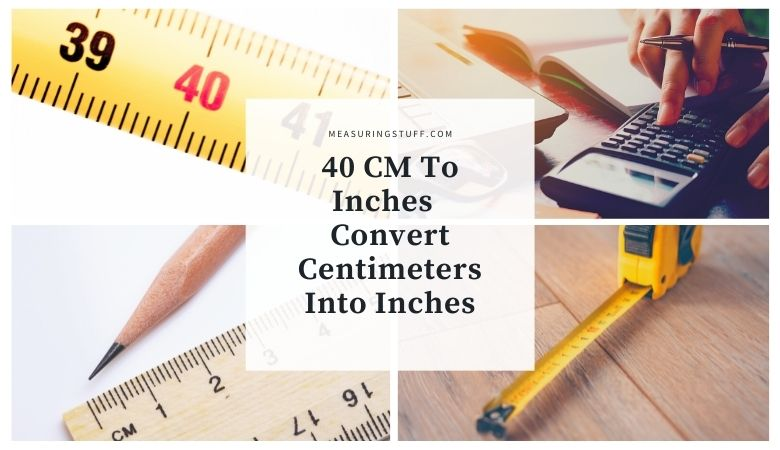# 40 CM To Inches – Convert Centimeters Into Inches

Converting centimeters into inches can be done easily by using a formula, using a conversion calculator, or using a quick reference chart. I will show you all 3 methods.

If you wish to convert 40 centimeters into inches, simply divide the number by 2.54.

For example, 40cm / 2.54 = 15.748 inches.

Regardless of how many centimeters you are looking to convert into inches, simply divide the number by 2.54.

If you wish to convert inches into centimeters, multiply the number by 2.54.

For example, 40 inches x 2.54 = 101.6 centimeters.

## Conversion calculator

Converting 40 centimeters into inches can also be done by using the following calculator.

Select length as the unit type, input 40 for no. of units, select centimeters for convert from, select inches for convert to, then click convert.

The calculator will give you the answer of 15.748.

## Conversion chart

You can also use this quick reference chart to convert centimeters into inches. Referencing 40 centimeters, you will see the conversion to inches.

## What is a centimeter?

According to Oxford Languages, a centimeter is a metric unit of length, equal to one-hundredth of a meter.

## What is an inch?

According to Oxford Languages, an inch is a unit of linear measure equal to one-twelfth of a foot (2.54 cm).

## What does 40 centimeters look like?

To get a visual reference of what 40 centimeters looks like, you can look at a standard ruler. The ruler has a length of 30 centimeters or 12 inches.

So by adding another third onto a standard ruler gives you exactly 40 centimeters. The ruler has both centimeters and inches measurements which makes it easy to convert between the two.

For our complete section of unit conversions including tables and calculators click the link here.